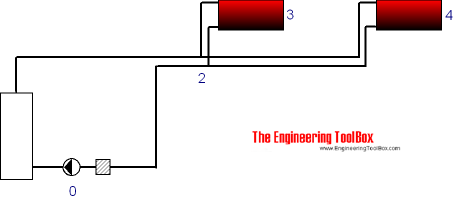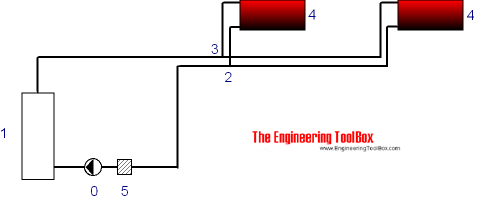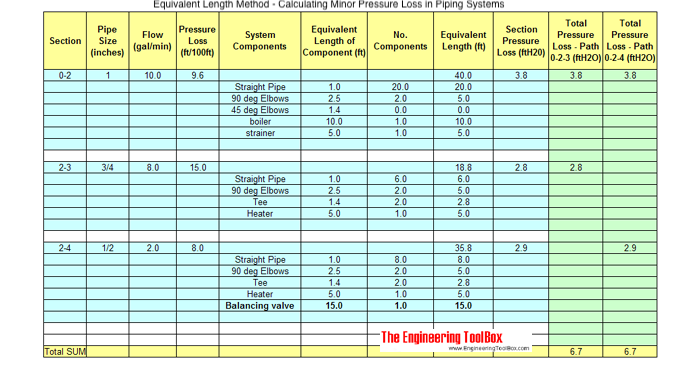Engineering ToolBox - Resources, Tools and Basic Information for Engineering and Design of Technical Applications!

# Equivalent Length Method - Minor Pressure Loss in Piping Systems

## Calculate minor pressure loss in piping systems with the Equivalent Pipe Length Method.

An efficient and simple way to calculate the pressure loss in a piping system is the "Equivalent Pipe Length Method".

### 1. Make a Diagram of the Piping System

Make a diagram where the system is structured with nodes as shown below.In the very simply circulating system used in this example the first node (0) is the pump. The next node is the tees (2) where the piping system splits up. The other nodes are the heating radiators.

In many systems the structure can be simplified as above since the piping in both directions are of same size. If a more fine grained approach is required, additional nodes can be added in both directions as shown below.### 2. Make a calculation table

With the most simplified nodes structure above a calculation table can be made as shown below. Each section from node to node is calculated by supplying length, volume flow, pipe size, pressure loss from diagrams or tables for the actual pipes and components in the sections and their individual equivalent length substituting the the minor loss.Note! The flow and pressure units must be adjusted to the data available for your piping system.

### 3. Add Volume flow, Pipe Size and Pressure Loss for each Section

Add actual pipe size and in each section. Use tabulated data or a diagrams.

The pressure loss may alternatively be calculated with the Hazen-Williams Equation or the Darcy-Weisbach Formula.

• Pressure loss for many types of pipes can be found here.

### 4. Add Equivalent Length of all Valves, Fittings and Straight Pipes

Add the equivalent length of all valves, components, fittings and straight pipes in the sections.

### 5. Summarize the Pressure Loss in each Section

Calculate and summarize the pressure loss in each section.

### 6. Summarize the Pressure Loss in all Paths

Finally, add up the pressure loss in all sections that form unique paths. In the example above there are two unique paths - one is section 0 - 2 - 3, the other is section 0 - 2 - 4. Add extra columns for additional paths in more complicated systems.

The highest pressure loss determines the pump head.

Add valves where it is necessary to balance the system. In the example above a balancing valve is added in section 2-4.

Note! The Equivalent Pipe Length Method can be adapted to most piping systems - like water supply systems, gravity heating systems and similar.

## Related Topics

• ### Design Strategies

Piping systems design strategies - documentation, P&ID, flow diagrams - capacities and limits.
• ### Fluid Flow and Pressure Loss

Pipe lines - fluid flow and pressure loss - water, sewer, steel pipes, pvc pipes, copper tubes and more.
• ### Heating

Heating systems - capacity and design of boilers, pipelines, heat exchangers, expansion systems and more.

## Related Documents

• ### Copper Tubes - Equivalent Length Pressure Loss in Fittings and Valves

Copper tube fittings and equivalent lengths - in feets of straight tube.
• ### Darcy-Weisbach Equation - Major Pressure and Head Loss due to Friction

The Darcy-Weisbach equation can be used to calculate the major pressure and head loss due to friction in ducts, pipes or tubes.
• ### Duct Sizing - the Equal Friction Method

The equal friction method for sizing air ducts is easy and straightforward to use.
• ### Equivalent Diameter - Rectangular vs. Circular HVAC Ducts

Rectangular equivalent diameter for air flows between 100 - 50000 cfm.
• ### Fluid Flow - Equivalent Diameter

Convert rectangular and oval duct geometry to a equivalent circular diameter - online calculator with imperial and SI-units.
• ### Hazen-Williams Friction Loss Equation - calculating Head Loss in Water Pipes

Friction head loss (ftH2O per 100 ft pipe) in water pipes can be estimated with the empirical Hazen-Williams equation.
• ### Hot Water Heating Systems - Online Design Application

Free online design tool for designing hot water heating systems - metric units.
• ### Hot Water Heating Systems - Online Design Application, Imperial Units

Online design tool for hot water heating systems.
• ### Hot Water Systems - Equivalent Length vs. Fittings Resistance

Equivalent length of fittings like bends, returns, tees and valves in hot water heating systems - equivalent length in feet and meter.
• ### Pipe and Tube System Components - Minor (Dynamic) Loss Coefficients

Minor loss coefficients for components used in pipe and tube systems.
• ### Pipe Lengths

Pipe is supplied and refered to as single random, double random or cut lengths
• ### PVC Pipes - Equivalent Length and Pressure Loss in Fittings

Minor loss in PVC and CPVC fittings expressed as equivalent length of straight pipe.
• ### PVC Pipes Schedule 80 - Friction Loss vs. Water Flow

Water flow in thermoplastic PVC and CPVC pipes Schedule 80 - friction loss (ft/100 ft, psi/100 ft) and flow velocities at dimensions ranging 1/2 to 16 inches.

## Engineering ToolBox - SketchUp Extension - Online 3D modeling!

Add standard and customized parametric components - like flange beams, lumbers, piping, stairs and more - to your Sketchup model with the Engineering ToolBox - SketchUp Extension - enabled for use with older versions of the amazing SketchUp Make and the newer "up to date" SketchUp Pro . Add the Engineering ToolBox extension to your SketchUp Make/Pro from the Extension Warehouse !

We don't collect information from our users. More about

## Citation

• The Engineering ToolBox (2004). Equivalent Length Method - Minor Pressure Loss in Piping Systems. [online] Available at: https://www.engineeringtoolbox.com/equivalent-pipe-length-method-d_804.html [Accessed Day Month Year].

Modify the access date according your visit.

9.29.12